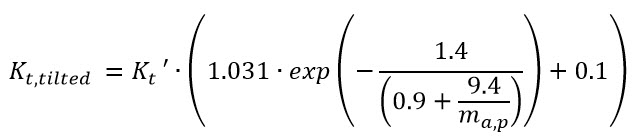# GTI DIRINT

This function derives direct normal irradiance (GB,n) and diffuse horizontal irradiance (GD) from the global tilted irradiance (GTi) using modifications to the DIRINT decomposition model and Perez transposition model. This allows for GTi to be input into an energy prediction, largely avoiding decomposition and transposition model errors (with the exception of calculations that require the direct/diffuse components, i.e. shading and IAM).

## Inputs## Outputs## Algorithm

This model implementation calculates a solution for incidence angles (θ) over and under 90° separately to allow solutions at times when the GTi measurement does not include any component of GD.

### 1. GTI DIRINT model for θ < 90°

Note that this model uses a modified (tilted) clearness index as described in the Clearness Index section. Unmodified DIRINT equations (see DIRINT Model) are used to calculate GB,n. GD can then be calculated using:Known errors are introduced when using the GTi as an input into the GTI DIRINT model (i.e. differences in proportions of diffuse and direct irradiance between global horizontal irradiance and GTi and the fact that GTi also includes ground reflected radiation). So, after Gand GB,n are calculated from the measured GTi using the reverse decomposition of GTI DIRINT, the forward transposition using Perez is used to calculate GTi as a check. The measured GTi and calculated GTi can then be compared and used as the iteration criteria in an optimization loop.

In the optimization loop, the initial calculation uses the measured GTi to calculate Kt. Subsequent iterations adjust the GTi until the Kt provides a GB,n and Gvalue such that a modeled GTi matches the measured GTi. The GTi value is adjusted using:Where i is the index corresponding to the iteration number, D is the difference between the modeled and measured GTi, and C is a coefficient to ensure an iterative solution (1.0 for i <3, 0.5 for 3 ≤ i < 10, 0.25 for 10 ≤ i < 20, and 0.125 for 20 ≤ i < 30). Often, only two iterations are needed. The following illustrates the flow chart for the model when θ < 90°.

##2. GTI DIRINT model for θ ≥ 90°

After GB,n and Gare calculated for θ < 90°, average early morning and late afternoon Kt‘ values are determined by averaging Kt‘ values for morning and afternoon instances when θ is between 65° and 85°. Besides Kt‘, the solution for GB,n requires Kt which is rearranged from the original DIRINT equation to solve for Kt instead of Kt‘:The Gcan then be determined using:## Reference

Marion, B., A model for deriving the direct normal and diffuse horizontal irradiance from the global tilted irradiance.  Solar Energy 122 (2015), pp. 1037-1046.